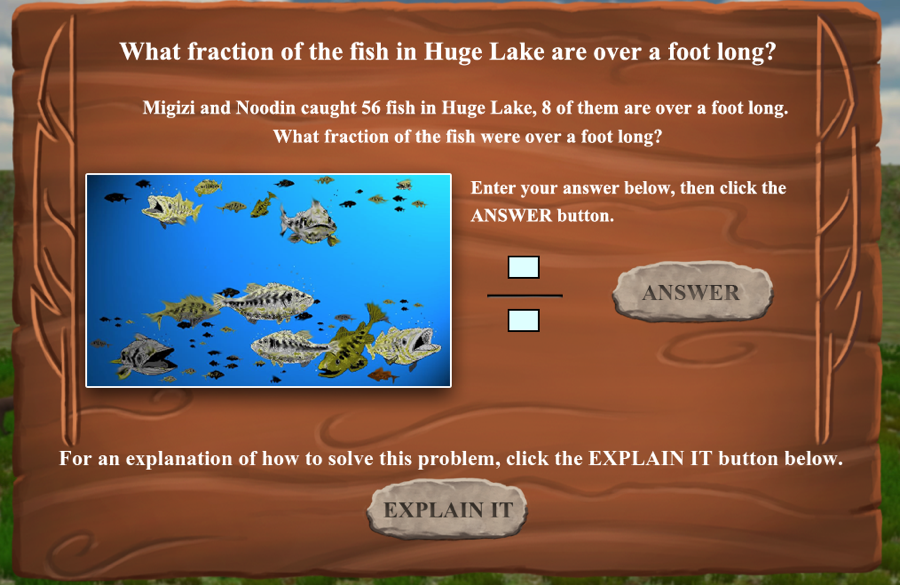# Fish Lake Game Standards

## Standard

Correctly identify the fraction 1/8 Understand a fraction 1/b as the quantity formed by 1 part when a whole is partitioned into b equal parts;
Correctly identify the fraction 2/8 Understand a fraction a/b as a multiple of 1/b
Compare 2/8 and 1/4 Compare two fractions with different numerators and different denominators
Correctly identify the fraction 25/125 OR 1/5 Understand a fraction a/b as a multiple of 1/b
Compare 2/8 and 25/125 Compare two fractions with different numerators and different denominators
Put in order fractions 1/4, 1/2, 3/4 Understand a fraction as a number on the number line; represent fractions on a number line diagram.
Subtract (5/8 + 2/8) from the whole to equal 1/8
• Solve word problems involving addition and subtraction of fractions referring to the same whole and having like denominators
• Express whole numbers as fractions, and recognize fractions that are equivalent to whole numbers
Solve for 1/2= 3/6 Recognize and generate simple equivalent fractions
Identify the fraction 8/56 Understand a fraction a/b as a multiple of 1/b
Solve for 20/50 as an equivalent fraction to 2/5 Recognize and generate simple equivalent fractions
Correctly identify the fraction 6/6
• Understand a fraction a/b as a multiple of 1/b
• Express whole numbers as fractions, and recognize fractions that are equivalent to whole numbers
Solve 1 1/2 + 3 1/2 = 5 Add and subtract mixed numbers with like denominators
Recognize that 3/4 of a smaller container is not equal to 3/4 of a large container Recognize that comparisons are valid only when the two fractions refer to the same whole.
Identify 6/36 on a number line, then subtract from 36/36
• Understand a fraction as a number on the number line
• Solve word problems involving addition and subtraction of fractions referring to the same whole and having like denominators, e.g., by using visual fraction models and equations to represent the problem
Solve 14 x 5/8 = 70/8. Then find the two whole numbers it falls between. Solve word problems involving multiplication of a fraction by a whole number
Reduce 36/27 to lowest terms as an improper fraction, then convert to a mixed number Recognize and generate equivalent fractions
Identify the fraction 4/7 then subtract 4/7 from 1. Solve word problems involving addition and subtraction of fractions referring to the same whole and having like denominators, e.g., by using visual fraction models and equations to represent the problem
Solve for 2/8 = 1/4 Recognize and generate simple equivalent fractions

# Fish Lake Problems

As students travel through a virtual world, they are presented with word problems using fractions. For example, if you are fishing for pike, what fraction of the fish shown in this lake are pike? A picture of a pike is given, so we know if a student gets it wrong it is not because he or she doesn't know what a pike looks like. There are three types of problems:

• Students are required to enter a number as an answer, usually in the form of a numerator and denominator, as shown in the first example below. For these problems, reports will show the exact answer the student entered, as well as if he or she is incorrect.
• Students are required to drag items to show a fraction on a number line. For these, teacher reports show the answer as correct or incorrect.
• Students are required to make a judgement, such as whether 3/4 of a small cart is the same size of 3/4 of a larger cart. For these, teacher reports show the answer as correct or incorrect.

A screenshot from the first type of problem is shown below.# Reading the Fish Lake Reports

The first line of the report gives the student username and the number of problems he or she attempted. This number may be more than 18 because a student may answer incorrectly on the first attempt and the answer correctly a second time.
• PROBLEM: The first column gives a short description of what the student is required to do. For example, in the problem above, the description is "Identify the fraction 8/56"
• CORRECT: Whether or not the student answered this question correctly.
• STUDENT ANSWER: Answer given by student, either the numeric answer or, in the case of multiple choice and drag-and-drop problems, whether the student answered correctly or incorrectly
• TIMES ATTEMPTED: The number of times a student attempted to answer the problem. A student may have one or more times answered incorrectly and then a correct answer.